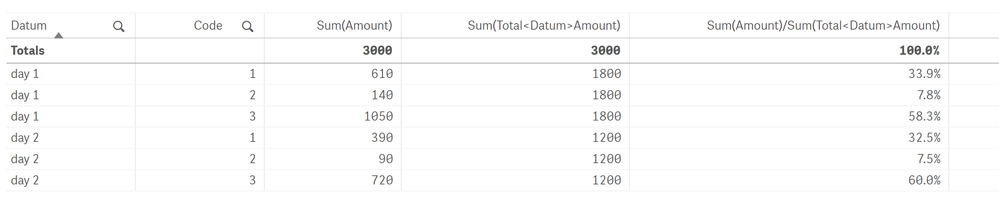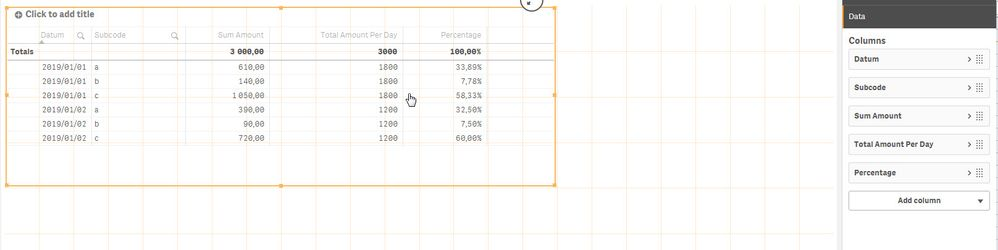# New to Qlik Sense

Discussion board where members can get started with Qlik Sense.

Announcements
Talk to Experts, a LIVE Q&A Webinar. Bring your Qlik Sense Business questions on Aug. 4th. Register
cancel
Showing results for
Did you mean:
HighlightedContributor

## Get a percentage per subcode per day

Hi Qlik Community,

This forum helped me out with quite a lot of questions already. I found numerous times someone else in the world facing the same challenge as I did and then another one who knew how to handle the challenge. However, this time my question is quite specific and I've been trying to look for an existing question & answer but I couldn't find one.

Hope someone can help me out since I'm stuck

So here is my problem:

I have 2 tables, one knowledge table with codes, subcodes and a percentage of how much of the code is the subcode. This is a given.

Code / Subcode / %

 1 a 50% 1 b 20% 1 c 30% 2 b 10% 2 c 90% 3 a 40% 3 c 60%

So multiple subcodes can be within one code and the subcodes also occur in different codes. a in code 1 is the same as a in code 3.

I also have my results per code, per day:

Datum / Code / Amount

 day 1 1 500 day 1 2 400 day 1 3 900 day 2 1 300 day 2 2 300 day 2 3 600

My desired outcome is:

Datum / Subcode / Amount / Total amount (per day) / Percentage subcode

 day 1 a 610 1800 33,9% day 1 b 140 1800 7,8% day 1 c 1050 1800 58,3% day 2 a 390 1200 32,5% day 2 b 90 1200 7,5% day 2 c 720 1200 60,0%

So all percentages add up to 100% per day.

I tried things like...

(avg(percentage)*sum(amount)) / sum(total <Datum> amount)

or

(sum(percentage)*sum(amount))/sum(total <Datum> amount)

but this doesn't work.

Hope someone can help me, any response is very much appreciated!

I added the example in excel

Labels (4)

• ### Total

3 Replies
HighlightedPartner

## Re: Get a percentage per subcode per day

You are almost there use below expressions

Sum per day - Sum(Total<Datum>Amount)

Percentage  Sub Code per day - Sum(Amount)/Sum(Total<Datum>Amount)

resultHighlightedMVP

## Re: Get a percentage per subcode per day

Try this

``=Sum(Amount*%)/Sum(TOTAL <Datum> Amount*%)``

HighlightedPartner

## Re: Get a percentage per subcode per day

Hi

If you add the two tables Table1 (Code, SubCode, %) and Table2 (Datum, Code, Amount) and link them by code you can create a table in Qlik Sense that looks like this as show in the screen shot belowThe formula for Sum Amount is  =Sum)Amount*%)

The formula for Total Amount Per Day is Sum(TOTAL <Datum> Amount*%)

The Formula for Percentage is =Sum(Amount*%)/Sum(TOTAL <Datum> Amount*%)

This results will show the same information as in you excel sheet.

Regards

Frank